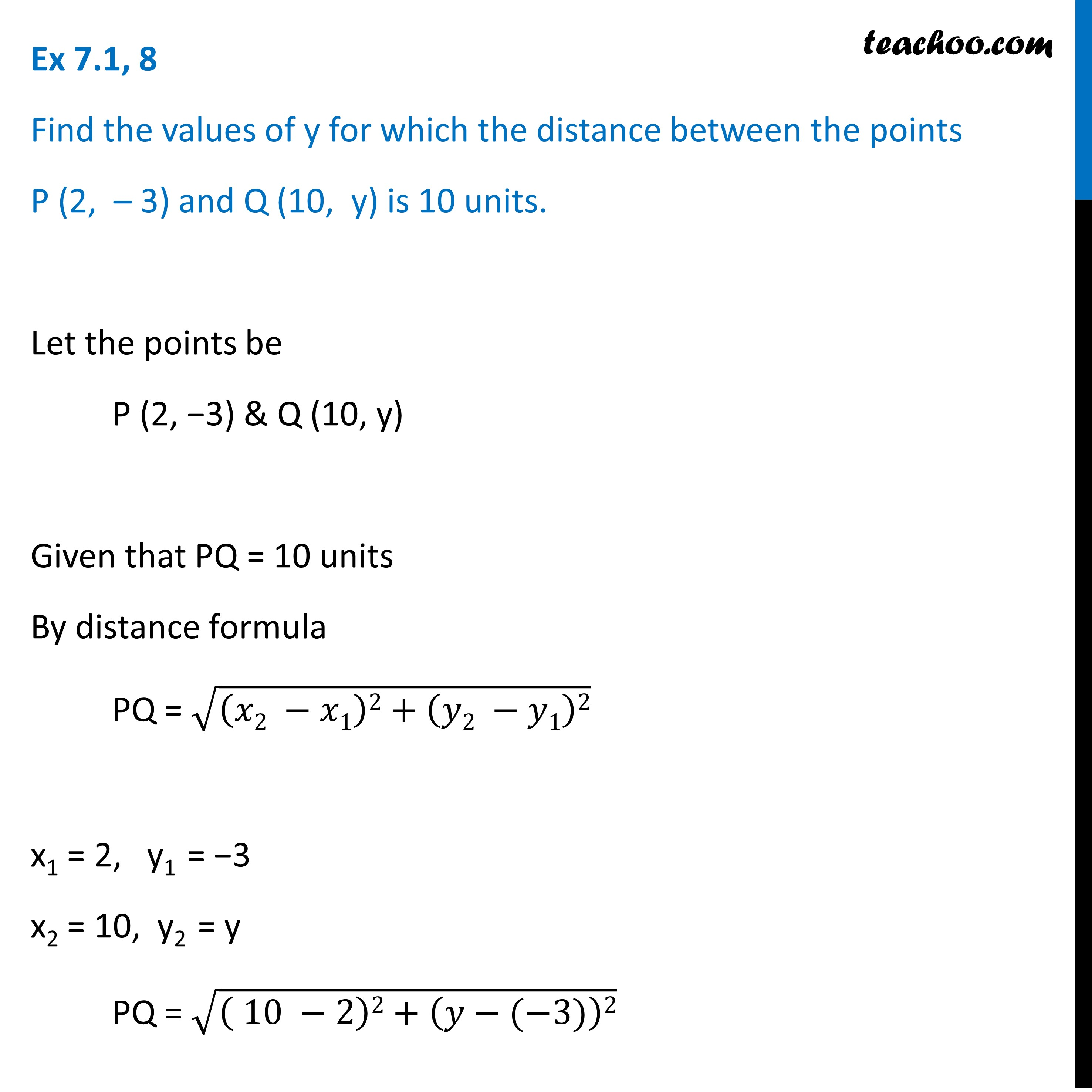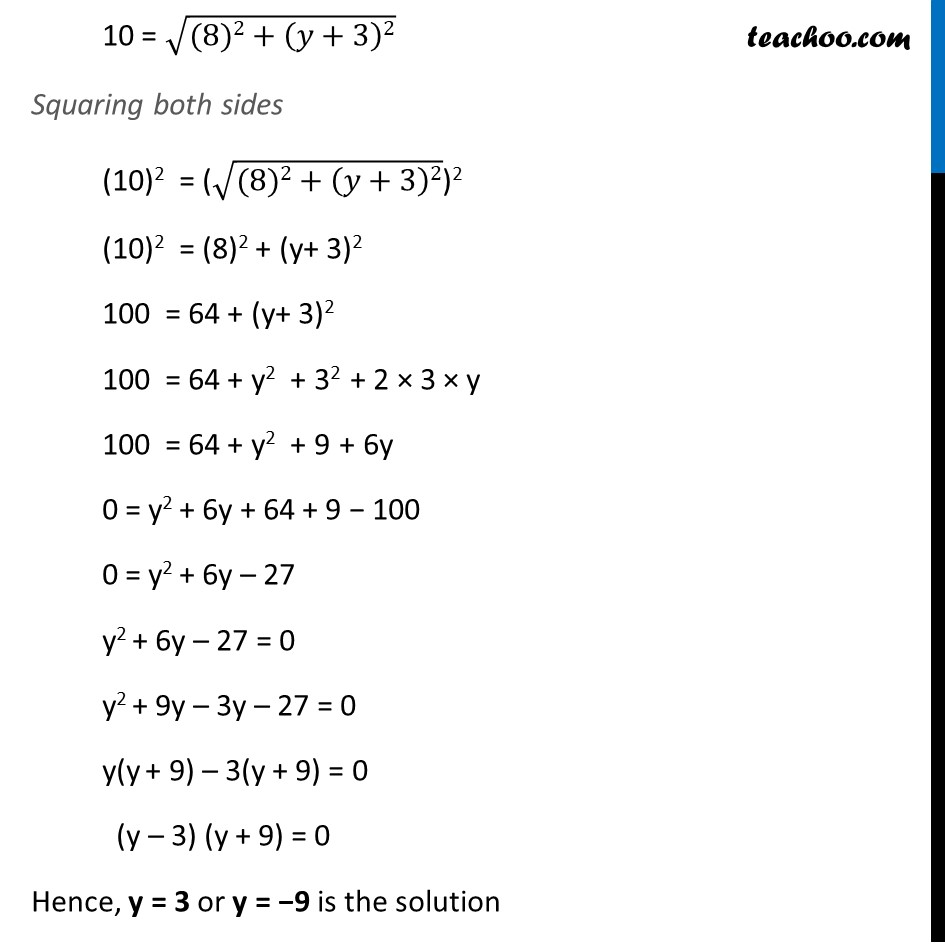Ex 7.1

Chapter 7 Class 10 Coordinate Geometry (Term 1)
Serial order wise983 students joined Teachoo Black. What are you waiting for?

### Transcript

Ex 7.1, 8 Find the values of y for which the distance between the points P (2, – 3) and Q (10, y) is 10 units. Let the points be P (2, – 3) & Q (10, y) Given that PQ = 10 units By distance formula PQ = √((𝑥2 −𝑥1)2+(𝑦2 −𝑦1)2) x1 = 2, y1 = −3 x2 = 10, y2 = y PQ = √(( 10 −2)2+(𝑦−(−3))2) 10 = √((8)2+(𝑦+3)2) Squaring both sides (10)2 = (√((8)2+(𝑦+3)2))2 (10)2 = (8)2 + (y+ 3)2 100 = 64 + (y+ 3)2 100 = 64 + y2 + 32 + 2 × 3 × y 100 = 64 + y2 + 9 + 6y 0 = y2 + 6y + 64 + 9 − 100 0 = y2 + 6y – 27 y2 + 6y – 27 = 0 y2 + 9y – 3y – 27 = 0 y(y + 9) – 3(y + 9) = 0 (y – 3) (y + 9) = 0 Hence, y = 3 or y = −9 is the solution